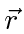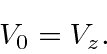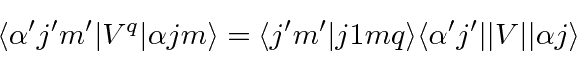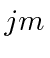## Vector Operators and the Wigner Eckart Theorem

There are some general features that we can derive about operators which are vectors, that is, operators that transform like a vector under rotations. We have seen in the sections on the Electric Dipole approximation and subsequent calculations that the vector operatorcould be written as its magnitudeand the spherical harmonics. We found that thecould change the orbital angular momentum (from initial to final state) by zero or one unit. This will be true for any vector operator.

In fact, because the vector operator is very much like adding an additionalto the initial state angular momentum, Wigner and Eckart proved that all matrix elements of vector operators can be written as a reduced matrix element which does not depend on any of the, and Clebsch-Gordan coefficients. The basic reason for this is that all vectors transform the same way under rotations, so all have the same angular properties, being written in terms of the.

Note that it makes sense to write a vectorin terms of the spherical harmonics usingandWe have already done this for angular momentum operators.

Lets consider our vectorwhere the integerruns from -1 to +1. The Wigner-Eckart theorem saysHererepresents all the (other) quantum numbers of the state, not the angular momentum quantum numbers.represent the usual angular momentum quantum numbers of the states.is a reduced matrix element. Its the same for all values ofand. (Its easy to understand that if we take a matrix element ofit will be 10 times the matrix element of. Nevertheless, all the angular part is the same. This theorem states that all vectors have essentially the same angular behavior. This theorem again allows us to deduce that.

The theorem can be generalized for spherical tensors of higher (or even lower) rank than a vector.

Jim Branson 2013-04-22